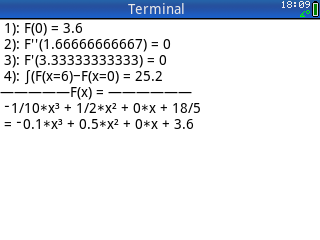## Function Defined by Preset Conditions

Description:Finds for a function f(x) of n-th degree ( n= 3 or 4 ) given as f(x) =*x^n + b*x^(n-1) + c*x^(n-2) + … the numerical values of the unknown coefficients a, b, c, … owing to preset conditions. These conditions may be: a given point P(x|y) as part of the graph of the function f(x), extremum or tangent in x for f´(x), point of inflexion in x as f´´(x)=- ( f´´(x)<>0 also possible ) - or the integral I (x) =nInt( f(x), x_lower, x_upper) between two points. After calculation, the graph F1(X) of the function is plotted.
Filename:findfunc.zip
ID:8810
Current version:1.3
Author:Claus Martin Dachselt
Size on calculator:7 KB
Platforms:Prime
User rating:Not yet rated (you must be logged in to vote)
Primary category:Math
Languages:ENG
File date:2018/08/15 10:47:46
Creation date:2018/08/15
Source code:Not included
Version history:2018/08/18: Updated to version 1.3
2018/04/09: Updated to version 1.2
Screenshot: